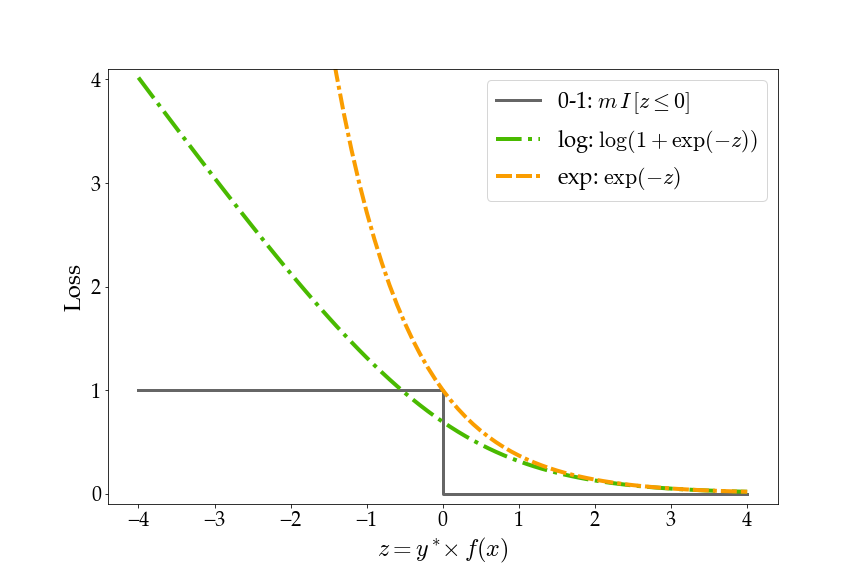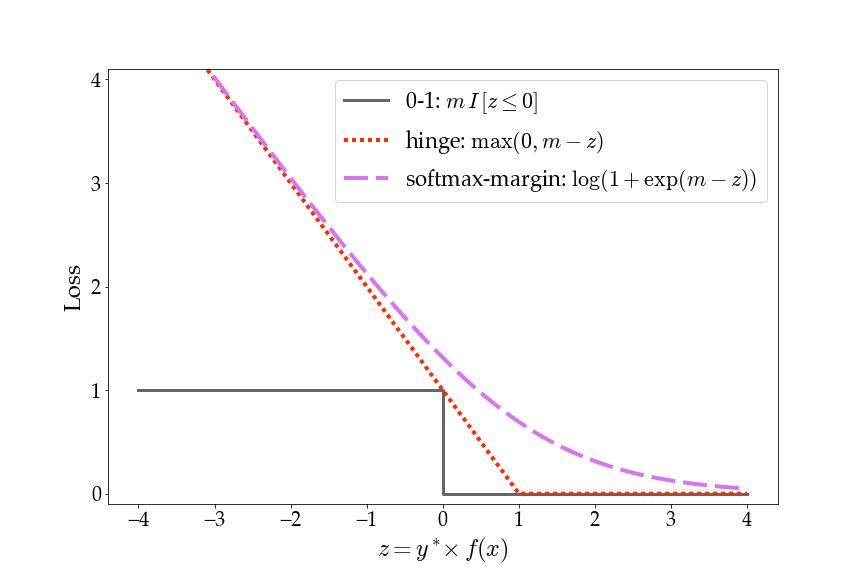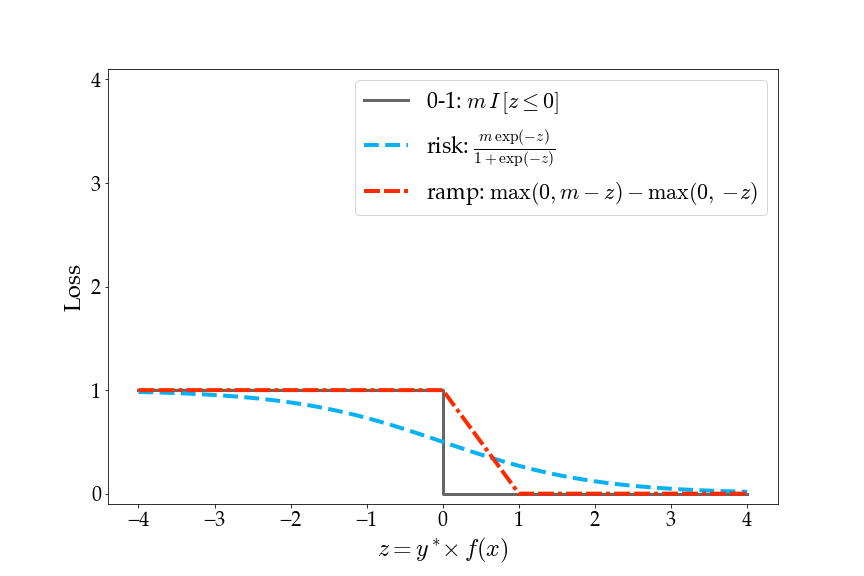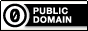Visualizing Losses for Binary Classification

This page hosts a Jupyter Notebook for plotting loss functions for binary classification.

The horizontal axis, z, represents the ground truth output y* multiplied by the classifier score f(x) for an input x. Since this is binary classification, y* is either -1 or 1 and f(x) is a scalar.All materials on this page are released under CC0 1.0 Universal (public domain).